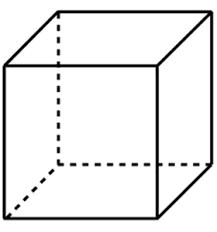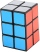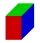# Cuboid and ratio

Cuboid has dimensions in ratio 1:2:6 and the surface area of the cuboid is 1000 dm2. Calculate the volume of the cuboid.

V =  1500 dm3

### Step-by-step explanation:Did you find an error or inaccuracy? Feel free to write us. Thank you!Tips to related online calculators
Check out our ratio calculator.
Tip: Our volume units converter will help you with the conversion of volume units.

## Related math problems and questions:

• Cuboid - ratioFind the volume of a block whose dimensions are in the ratio 2: 3: 4 and the surface is 117 dm2.
• 3rd dimensionThe block has a surface of 42 dm2 and its dimensions are 3 dm and 2 dm. What is the third dimension?
• Volume and surfaceCalculate the volume and surface area of the cylinder when the cylinder height and base diameter is in a ratio of 3:4 and the area of the cylinder jacket is 24 dm2.
• Cuboid edgesThe lengths of the cuboid edges are in the ratio 2: 3: 4. Find their length if you know that the surface of the cuboid is 468 m2.
• Cuboid - ratiosThe sizes of the edges of the cuboid are in the ratio 2: 3: 5. The smallest wall has an area of 54 cm2. Calculate the surface area and volume of this cuboid.
• Ratio-cuboidThe lengths of the edges of the cuboid are in the ratio 2: 3: 6. Its body diagonal is 14 cm long. Calculate the volume and surface area of the cuboid.
• PrismCalculate the height of the prism having a surface area 448.88 dm² wherein the base is square with a side of 6.2 dm. What will be its volume in hectoliters?
• Cuboid - edgesThe cuboid has dimensions in ratio 4: 3: 5, the shortest edge is 12 cm long. Find: (A) the lengths of the remaining edges, (B) the surface of the cuboid, (C) the volume of the cuboid
• Cube wallsFind the volume and surface area of the cube if the area of one wall is 40cm2.
• The cubeThe cube has a surface area of 216 dm2. Calculate: a) the content of one wall, b) edge length, c) cube volume.
• Rectangular cuboidThe rectangular cuboid has a surface area 5334 cm2, and its dimensions are in the ratio 2:4:5. Find the volume of this rectangular cuboid.
• Surface of the cuboidFind the surface of the cuboid if its edges have the dimensions a, 2/3a, 2a
• Cuboid diagonalCalculate the volume and surface area of the cuboid ABCDEFGH, which sides a, b, c has dimensions in the ratio of 9:3:8. If you know that the diagonal wall AC is 86 cm, and the angle between AC and space diagonal AG is 25 degrees.
• Cuboid and ratioFind the dimensions of a cuboid having a volume of 810 cm3 if the lengths of its edges coming from the same vertex are in ratio 2: 3: 5
• Surface areaThe volume of a cone is 1000 cm3 and the content area of the axis cut is 100 cm2. Calculate the surface area of the cone.
• PoolHow many hl of water is in a cuboid pool (a = 25m, b = 8m) if the area of the wetted walls is 279.2 m2?
• Cuboid diagonalsThe cuboid has dimensions of 15, 20 and 40 cm. Calculate its volume and surface, the length of the body diagonal and the lengths of all three wall diagonals.